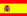You are here : > Home> Utilities > Mathematics & Statistics > Directly proportional division >

### Directly proportional division

A direct proportional division consists of dividing one number in parts, directly proportional. The higher the proportion, the greater the part.
For example: The division of household expenses in proportion to the earnings of participants:
Monthly costs = \$1,500.00; Net income of the participants: A = \$1,500.00; B = \$1,000.00; C = \$500.00;
N = Amount for the division = 1500;
Proportions (X): a = 1500; b = 1000; C = 500;
Solution:
Participants: A = \$750.00; B = \$500.00; C = \$250.00.
See full text Proportional Division
Enter the number and proportions, separated by commas. The decimal number is possible, but not the blank, zero or a negative number.
Use the point as decimal separator. Ex. For the number 5,152.47, enter 5152.47; The result is showed, automatically in the form, rounded, by clicking on "Calculate".

 Directly proportional division Number: Proportions (X): Number of parts: Proportional parts: